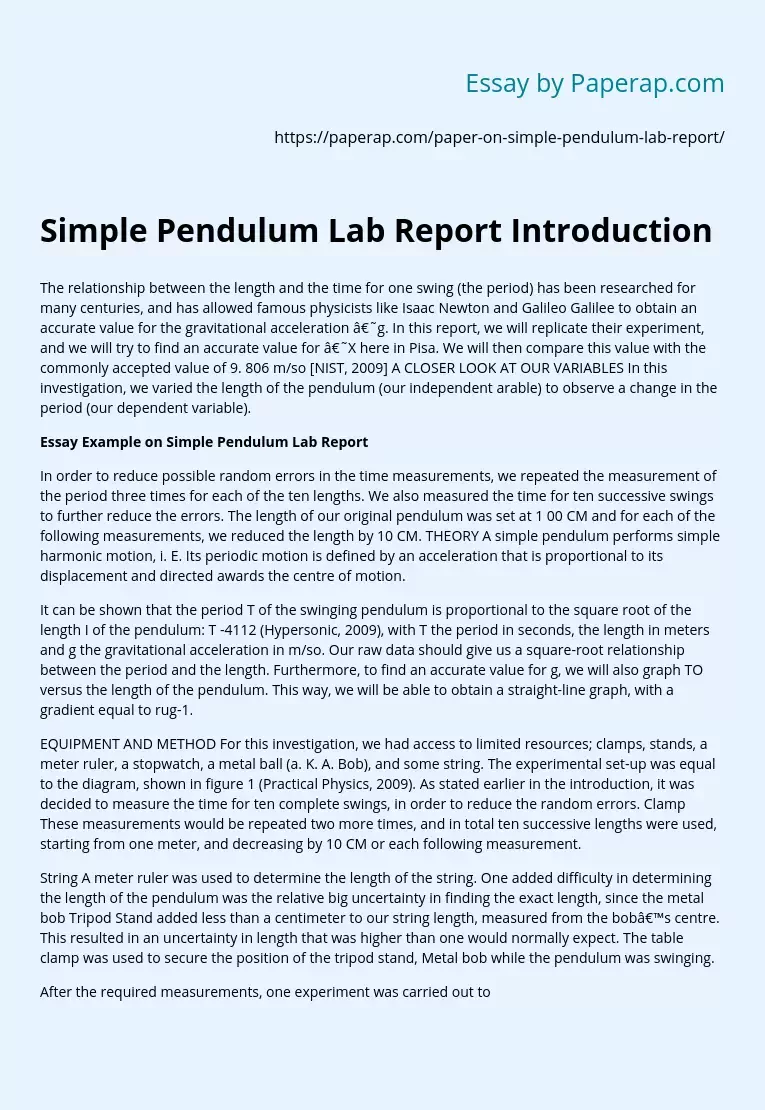# Simple Pendulum Lab Report Introduction

The relationship between the length and the time for one swing (the period) has been researched for many centuries, and has allowed famous physicists like Isaac Newton and Galileo Galilee to obtain an accurate value for the gravitational acceleration ‘g. In this report, we will replicate their experiment, and we will try to find an accurate value for ‘X here in Pisa. We will then compare this value with the commonly accepted value of 9. 806 m/so [NIST, 2009] A CLOSER LOOK AT OUR VARIABLES In this investigation, we varied the length of the pendulum (our independent arable) to observe a change in the period (our dependent variable).

## Essay Example on Simple Pendulum Lab Report

In order to reduce possible random errors in the time measurements, we repeated the measurement of the period three times for each of the ten lengths. We also measured the time for ten successive swings to further reduce the errors. The length of our original pendulum was set at 1 00 CM and for each of the following measurements, we reduced the length by 10 CM.

THEORY A simple pendulum performs simple harmonic motion, i. E. Its periodic motion is defined by an acceleration that is proportional to its displacement and directed awards the centre of motion.

It can be shown that the period T of the swinging pendulum is proportional to the square root of the length I of the pendulum: T -4112 (Hypersonic, 2009), with T the period in seconds, the length in meters and g the gravitational acceleration in m/so.

Get quality help nowBella HamiltonVerified

Proficient in: Experiment5 (234)

“ Very organized ,I enjoyed and Loved every bit of our professional interaction ”+84 relevant experts are online

Our raw data should give us a square-root relationship between the period and the length. Furthermore, to find an accurate value for g, we will also graph TO versus the length of the pendulum. This way, we will be able to obtain a straight-line graph, with a gradient equal to rug-1.

EQUIPMENT AND METHOD For this investigation, we had access to limited resources; clamps, stands, a meter ruler, a stopwatch, a metal ball (a. K. A. Bob), and some string. The experimental set-up was equal to the diagram, shown in figure 1 (Practical Physics, 2009). As stated earlier in the introduction, it was decided to measure the time for ten complete swings, in order to reduce the random errors. Clamp These measurements would be repeated two more times, and in total ten successive lengths were used, starting from one meter, and decreasing by 10 CM or each following measurement.

String A meter ruler was used to determine the length of the string. One added difficulty in determining the length of the pendulum was the relative big uncertainty in finding the exact length, since the metal bob Tripod Stand added less than a centimeter to our string length, measured from the bob’s centre. This resulted in an uncertainty in length that was higher than one would normally expect. The table clamp was used to secure the position of the tripod stand, Metal bob while the pendulum was swinging.

After the required measurements, one experiment was carried out to find the Figure 1: Diagram of the set-up for this experiment degree of damping in our set-up. Damping always occurs when there is friction, but exactly how significant the degree of damping in our experimental set-up was, remained uncertain. Depending on the degree of damping, it may or may not have a significant effect on our measurements. All measurements were taken under the same conditions, using the same metal bob, the same ruler, in the same room, and at approximately 26 degrees Celsius.